#### 期刊菜单

VHF频段极窄带高温超导滤波器的研制
Development of Very Narrow Band High Temperature Superconducting Filters in VHF Band
DOI: 10.12677/OJCS.2022.111001, PDF, HTML, XML, 下载: 167  浏览: 531

Abstract: In this paper, a fourth-order very narrow-band high-temperature superconducting filter with a relative bandwidth of 0.8‰ and insertion loss less than 0.7 dB is developed for the VHF band. The filter is based on a Chebyshev prototype design and is fabricated from DyBCO, a 2-inch diameter magnesium oxide (MgO) based high-temperature superconducting thin film material. The circuit construction and electromagnetic field simulation of the filter are performed by using Sonnet soft-ware. Equivalent circuit diagrams, theoretical curves, coupling matrices, physical circuits, simulations and practical test results of the very narrow band filter are presented in the article.

1. 引言

2013年天津海泰超导公司成功设计了一款相对带宽1‰的六阶极窄带宽高温超导滤波器 ，达到了高温超导滤波器窄带宽的最新高度。该文献中设计的中心频率约为1 GHz，属于超高频。2020年张戈戎等人完成了一款相对带宽为3.3‰的八阶窄带滤波器设计 ，可有效地提高系统的抗干扰性能。同年，杭州电子科技大学顾月等人成功实现了一款中心频率为45.5 MHz、相对带宽为2%的六阶高温超导窄带滤波器 ，为VHF频段的窄带滤波器提供了新的解决方案。以上文献均为高温超导滤波器可以实现的极窄带宽提供了实践基础。

VHF频段的工作频率较低，滤波器面积较大；带宽极窄，要求超导薄膜的均匀性好而且超导电路的加工误差低，增加了滤波器设计和制造的难度。本文的滤波器设计解决了这两个难点，采用均匀阻抗谐振器有效地降低滤波器的尺寸；采用电场耦合的方式可实现窄带宽的要求，采用多源热共蒸法制备高温超导薄膜提高了薄膜的均匀性以及利用半导体平面精细加工工艺制作微带电路，提高了物理电路制作的加工精度，以便将误差控制在可接受的范围内；成功研制了工作于VHF频段且相对带宽为0.8‰的四阶高温超导滤波器。

2. 理论计算

${K}_{i,i+1}=\frac{FBW}{\sqrt{{g}_{i}{g}_{i+1}}}$ (1)

${Q}_{ei}={Q}_{eo}=\frac{{g}_{i}}{FBW}$ (2)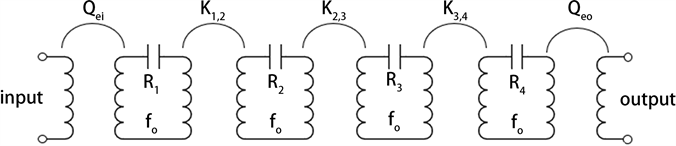Figure 1. Equivalent circuit diagram of fourth-order superconducting filter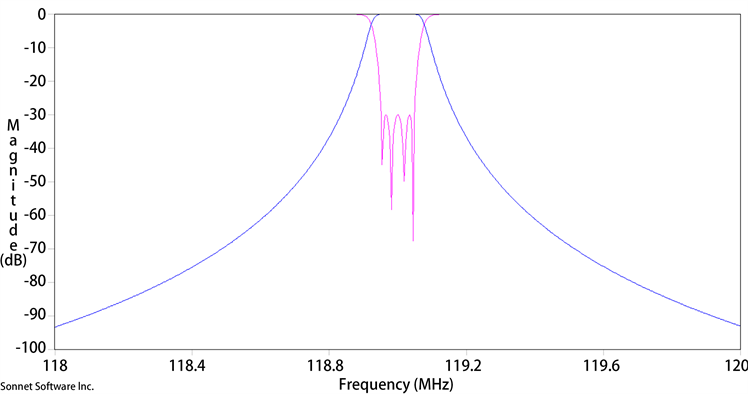Figure 2. Theoretical curve

$\left[\begin{array}{cccc}0& K1,2& 0& 0\\ K2,1& 0& K2,3& 0\\ 0& K3,2& 0& K3,4\\ 0& 0& K4,3& 0\end{array}\right]$

${K}_{1,2}={K}_{2,1}={K}_{3,4}={K}_{4,3}=0.000781054,$

${K}_{2,3}={K}_{3,2}=0.000703175,$

${Q}_{ei}={Q}_{eo}=1190.$

3. 滤波器的设计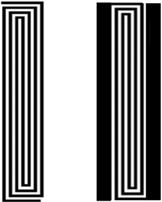(a) (b)

Figure 3. (a) Schematic diagram of UIR resonator structure; (b) Schematic diagram of SIR resonator structure

UIR结构谐振器长度计算公式如公式(3)，

$L=\frac{{\lambda }_{0}}{2}=\frac{c}{2{f}_{0}\sqrt{{\epsilon }_{eff}}}$ (3)

SIR结构谐振器的长度根据文献公式计算 ：

${\theta }_{T}={\theta }_{1}+{\theta }_{2}={\theta }_{1}+\mathrm{arctan}\left(K/\mathrm{tan}{\theta }_{1}\right)$ (4)

${L}_{n}=\frac{2{\theta }_{T}}{\text{π}}$ (5)

${k}_{i,i+1}=\frac{{f}_{1}^{2}-{f}_{2}^{2}}{{f}_{1}^{2}+{f}_{2}^{2}}$ (6)Figure 4. Comparison of coupling curves for the same spacing of SIR and UIR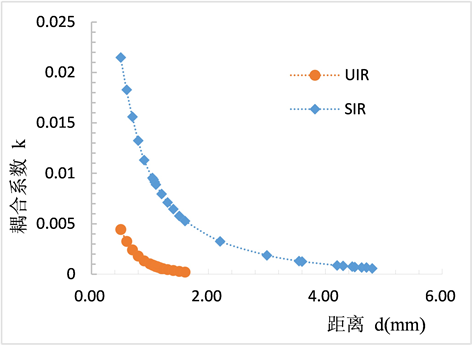Figure 5. Plot of adjacent resonator coupling coefficient versus spacing

${Q}_{e}=\frac{{f}_{0}}{B{W}_{-3\text{dB}}}$ (7)

Qe为谐振器输入输出端口的外部品质因数，f0为中心频率，BW−3dB是仿真曲线−3 dB处的带宽。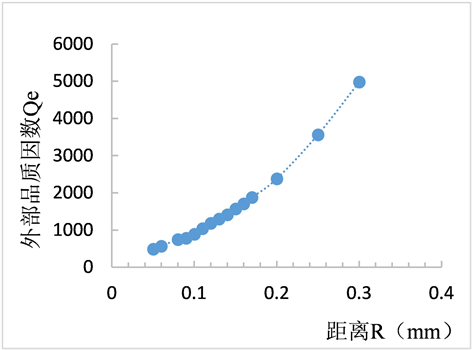Figure 6. Plot of external quality factor versus distance R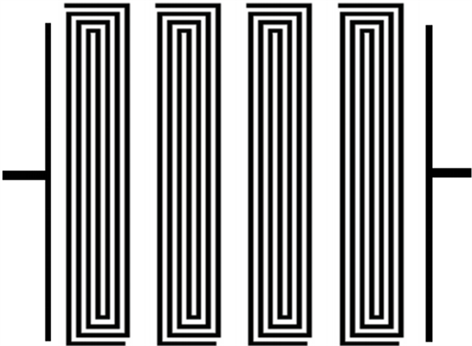Figure 7. Schematic diagram of high-temperature superconducting filter structure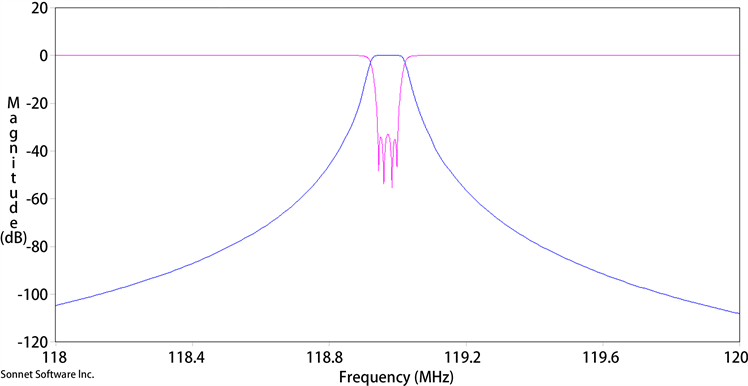Figure 8. High-temperature superconducting filter simulation curve

4. 滤波器测试结果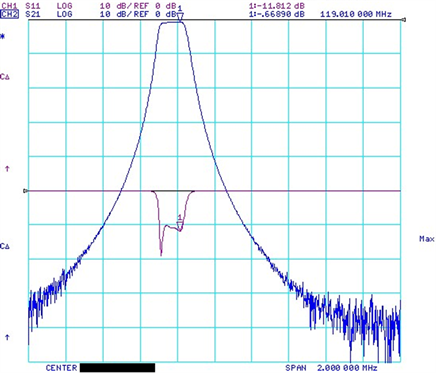Figure 9. Fourth-order superconducting filter test results

5. 结论

  Onnesh, K. (1911) The Resistance of Pure Mercury at Helium Temperatures. Communications Physical Laboratory University of Leiden, 12, 1.  Wu, M.K., Ashburn, J.R., Torng, C., Hor, P. H., Meng, R. L., Gao, L., et al. (1987) Superconductivity at 93 K in a New Mixed-Phase Y-Ba-Cu-O Compound System at Ambient Pressure. Physical Review Letters, 58, 908. https://doi.org/10.1103/PhysRevLett.58.908  胡来平, 张士刚, 张梅, 丁晓杰, 王生旺. 深空探测用X波段高带外抑制超导滤波器的研制[J]. 低温与超导, 2020, 48(7): 82-85. https://doi.org/10.16711/j.1001-7100.2020.07.017  季来运, 高文斌, 何立娟, 王朗, 孙钧, 李演奇. 六阶极窄带宽高温超导滤波器的研制[J]. 中国科学: 信息科学, 2013, 43(8): 1058-1064.  张戈戎. UHF频段无线收发组件的设计与仿真[D]: [硕士学位论文]. 淮南: 安徽理工大学, 2020.  顾月, 官伯然, 杜铁钧. 一种VHF高温超导窄带滤波器的研究[J]. 微波学报, 2020, 36(6): 61-63+69. https://doi.org/10.14183/j.cnki.1005-6122.202006013  Hong, J.S.G. and Lancaster, M.J. (2004) Microstrip Filters for RF/Microwave Applications. John Wiley & Sons, Hoboken.  Makimoto, M. and Yamashita, S. (1980) Bandpass Filters Using Parallel Coupled Stripline Stepped Impedance Resonators. IEEE Transactions on Microwave Theory and Techniques, 28, 1413-1417. https://doi.org/10.1109/TMTT.1980.1130258